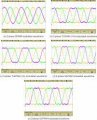# Frequecy and voltage control of VFDs using pwm technique

Thread Starter

#### ANIMO

Joined Sep 12, 2020
1
I had some doubt in PWM concepts, they are as follows
1) How to choose the switching frequency ( frequency of carrier signal ) so that the output voltage waveform of the inverter(which drives the induction motor) would have a fundamental frequency of 60hz/50hz.
2) Is the frequency of output dependant on the modulating signal frequency rather than the frequency of the carrier signal.
3) Is varying the modulating index the only way to control the output RMS voltage.

I am sorry for any grammatical mistakes as English is only my second language. Thank you for taking the time to read my queries.

#### MaxHeadRoom

Joined Jul 18, 2013
22,727
In your title you mention VFD's ?
Why do you need to use PWM?
The vast majority of VFD's use 0v-10dc to control RPM.
Some DIY CNC Software such as Mach etc, output a PWM signal that has to be converted to analogue in order to use it for VFD input.
Max.

#### TechWise

Joined Aug 24, 2018
151
I had some doubt in PWM concepts, they are as follows
1) How to choose the switching frequency ( frequency of carrier signal ) so that the output voltage waveform of the inverter(which drives the induction motor) would have a fundamental frequency of 60hz/50hz.
2) Is the frequency of output dependant on the modulating signal frequency rather than the frequency of the carrier signal.
3) Is varying the modulating index the only way to control the output RMS voltage.

I am sorry for any grammatical mistakes as English is only my second language. Thank you for taking the time to read my queries.
1. The minimum switching frequency is dictated by the quality of the output waveform that you want and the size of the filter that you are prepared to use. For a machine drive, the inductance of the machine windings is the filter. The maximum switching frequency is dictated by the type of switching device and how much switching loss you can cope with.
2. The carrier frequency and hence the switching frequency does not determine the output frequency. The output frequency will depend on the modulating signal.
3. Varying the modulation index will vary the output voltage magnitude, assuming the system does not become over-modulated. In theory, you could also adjust the output voltage by increasing or decreasing the DC link voltage but you would normally aim to have a constant DC voltage.

•ANIMO

#### nsaspook

Joined Aug 27, 2009
8,186
You can also inject a third harmonic to the sine modulation to increase DC link voltage utilization (output RMS voltage).

https://www.infineon.com/dgdl/AP160...M.pdf?fileId=db3a304412b407950112b40a1bf20453

If certain portion of the third harmonics wave is injected into the sine wave "a saddle” , the resulted modulated wave will appear as saddle-like shape (Figure 9) and the amplitude will obviously decrease. With keeping m<1, the amplitude of the fundamental wave can exceed that of the triangle wave and thus utilization ratio of the DC-bus voltage increases.https://www.ncbi.nlm.nih.gov/pmc/articles/PMC3231115/

3.5. Methods based on PWM strategies
There are many methods based on PWM control schemes, but the most relevant are conventional 120°, elimination of virtual neutral point, techniques for low speed, high speed and small power applications, and direct current controlled, which are explained below.